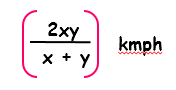# Average Quantitative Aptitude Interview Questions and Answers Part 1

## Average:

• Average means a number which is between the largest and the smallest number.
• The average is found by adding all the values in the set, then dividing the sum by the number of values.

## 1.Average Formula:## 2.Average Speed:

Suppose a man covers a certain distance at x kmph and an equal distance at y kmph.
Then, the average speed during the whole journey is3.A library has an average of 510 visitors on Sundays and 240 on other days. The average number of visitors per day in a month of 30 days beginning with a Sunday?